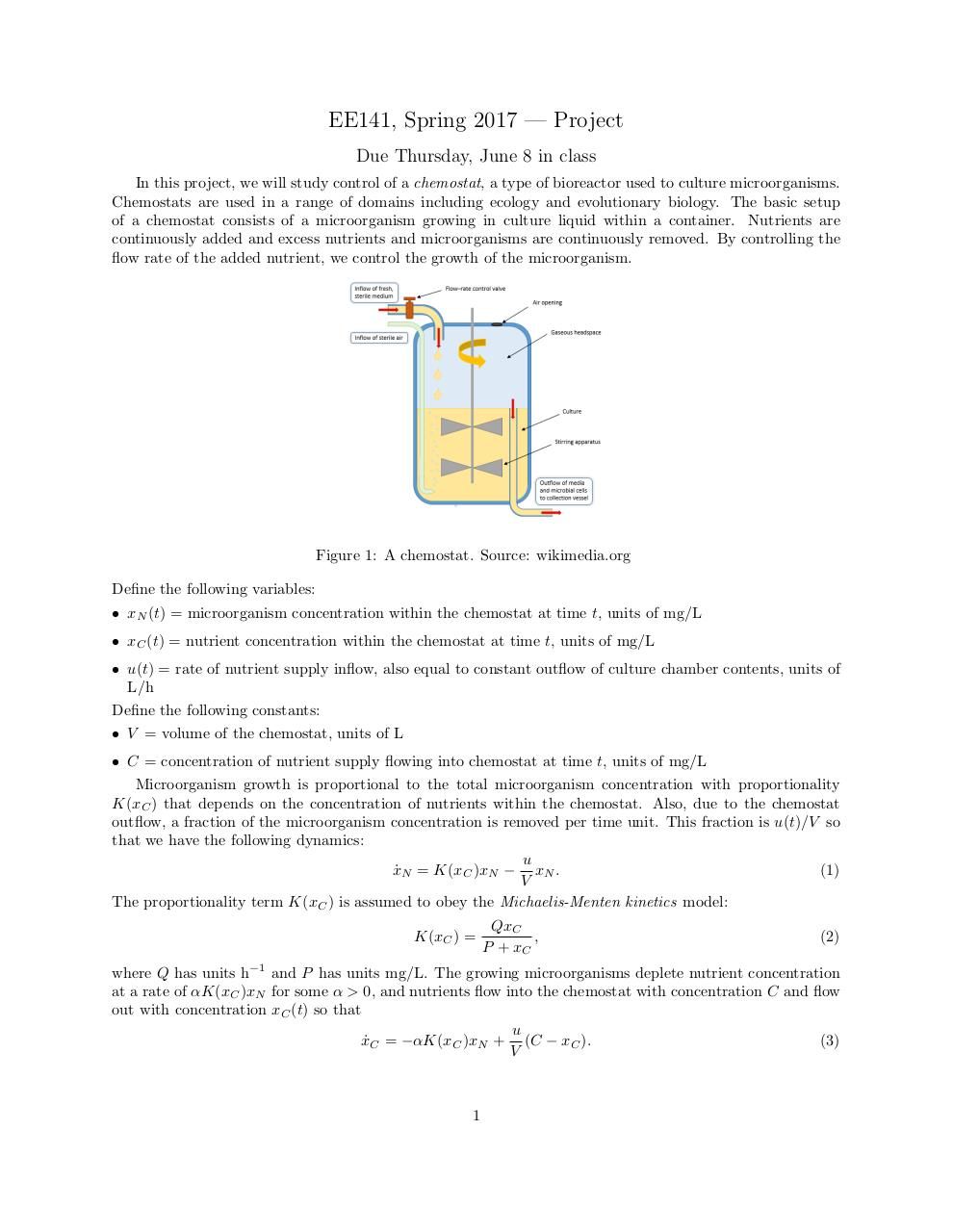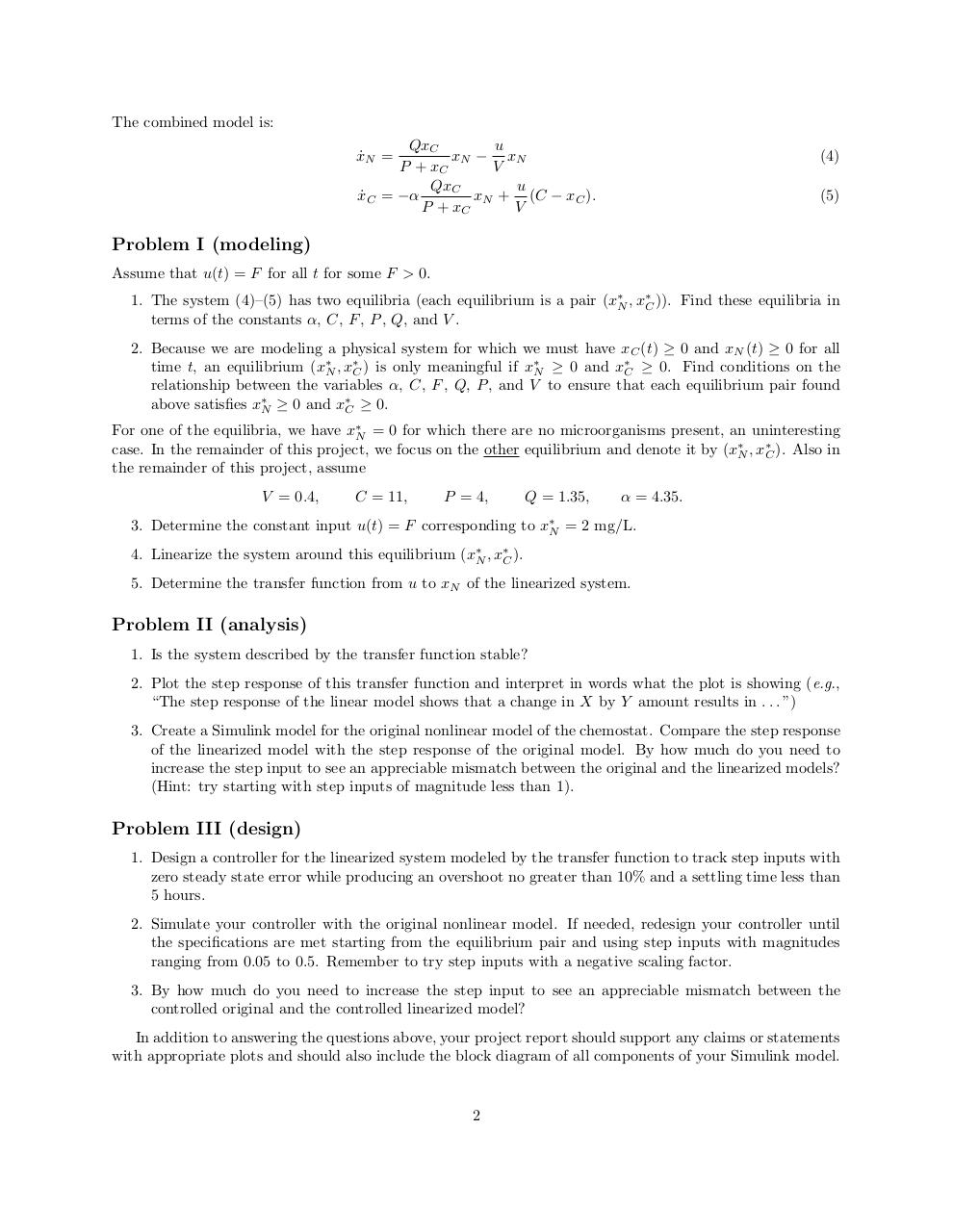# project uploaded .pdf

### File information

Original filename: project_uploaded.pdf

This PDF 1.5 document has been generated by TeX / pdfTeX-1.40.17, and has been sent on pdf-archive.com on 20/06/2017 at 04:21, from IP address 172.114.x.x. The current document download page has been viewed 241 times.
File size: 126 KB (2 pages).
Privacy: public file

## Download original PDF file

project_uploaded.pdf (PDF, 126 KB)

### Document preview

EE141, Spring 2017 — Project
Due Thursday, June 8 in class
In this project, we will study control of a chemostat, a type of bioreactor used to culture microorganisms.
Chemostats are used in a range of domains including ecology and evolutionary biology. The basic setup
of a chemostat consists of a microorganism growing in culture liquid within a container. Nutrients are
continuously added and excess nutrients and microorganisms are continuously removed. By controlling the
flow rate of the added nutrient, we control the growth of the microorganism.

Figure 1: A chemostat. Source: wikimedia.org
Define the following variables:
• xN (t) = microorganism concentration within the chemostat at time t, units of mg/L
• xC (t) = nutrient concentration within the chemostat at time t, units of mg/L
• u(t) = rate of nutrient supply inflow, also equal to constant outflow of culture chamber contents, units of
L/h
Define the following constants:
• V = volume of the chemostat, units of L
• C = concentration of nutrient supply flowing into chemostat at time t, units of mg/L
Microorganism growth is proportional to the total microorganism concentration with proportionality
K(xC ) that depends on the concentration of nutrients within the chemostat. Also, due to the chemostat
outflow, a fraction of the microorganism concentration is removed per time unit. This fraction is u(t)/V so
that we have the following dynamics:
u
x˙ N = K(xC )xN − xN .
(1)
V
The proportionality term K(xC ) is assumed to obey the Michaelis-Menten kinetics model:
K(xC ) =

QxC
,
P + xC

(2)

where Q has units h−1 and P has units mg/L. The growing microorganisms deplete nutrient concentration
at a rate of αK(xC )xN for some α &gt; 0, and nutrients flow into the chemostat with concentration C and flow
out with concentration xC (t) so that
u
x˙ C = −αK(xC )xN + (C − xC ).
(3)
V

1

The combined model is:
QxC
u
xN − xN
P + xC
V
u
QxC
xN + (C − xC ).
x˙ C = −α
P + xC
V

x˙ N =

(4)
(5)

Problem I (modeling)
Assume that u(t) = F for all t for some F &gt; 0.
1. The system (4)–(5) has two equilibria (each equilibrium is a pair (x∗N , x∗C )). Find these equilibria in
terms of the constants α, C, F , P , Q, and V .
2. Because we are modeling a physical system for which we must have xC (t) ≥ 0 and xN (t) ≥ 0 for all
time t, an equilibrium (x∗N , x∗C ) is only meaningful if x∗N ≥ 0 and x∗C ≥ 0. Find conditions on the
relationship between the variables α, C, F , Q, P , and V to ensure that each equilibrium pair found
above satisfies x∗N ≥ 0 and x∗C ≥ 0.
For one of the equilibria, we have x∗N = 0 for which there are no microorganisms present, an uninteresting
case. In the remainder of this project, we focus on the other equilibrium and denote it by (x∗N , x∗C ). Also in
the remainder of this project, assume
V = 0.4,

C = 11,

P = 4,

Q = 1.35,

α = 4.35.

3. Determine the constant input u(t) = F corresponding to x∗N = 2 mg/L.
4. Linearize the system around this equilibrium (x∗N , x∗C ).
5. Determine the transfer function from u to xN of the linearized system.

Problem II (analysis)
1. Is the system described by the transfer function stable?
2. Plot the step response of this transfer function and interpret in words what the plot is showing (e.g.,
“The step response of the linear model shows that a change in X by Y amount results in . . . ”)
3. Create a Simulink model for the original nonlinear model of the chemostat. Compare the step response
of the linearized model with the step response of the original model. By how much do you need to
increase the step input to see an appreciable mismatch between the original and the linearized models?
(Hint: try starting with step inputs of magnitude less than 1).

Problem III (design)
1. Design a controller for the linearized system modeled by the transfer function to track step inputs with
zero steady state error while producing an overshoot no greater than 10% and a settling time less than
5 hours.
2. Simulate your controller with the original nonlinear model. If needed, redesign your controller until
the specifications are met starting from the equilibrium pair and using step inputs with magnitudes
ranging from 0.05 to 0.5. Remember to try step inputs with a negative scaling factor.
3. By how much do you need to increase the step input to see an appreciable mismatch between the
controlled original and the controlled linearized model?
In addition to answering the questions above, your project report should support any claims or statements
with appropriate plots and should also include the block diagram of all components of your Simulink model.

2### Link to this page

#### Permanent link

Use the permanent link to the download page to share your document on Facebook, Twitter, LinkedIn, or directly with a contact by e-Mail, Messenger, Whatsapp, Line..

#### Short link

Use the short link to share your document on Twitter or by text message (SMS)

#### HTML Code

Copy the following HTML code to share your document on a Website or Blog

#### QR Code### Related keywords